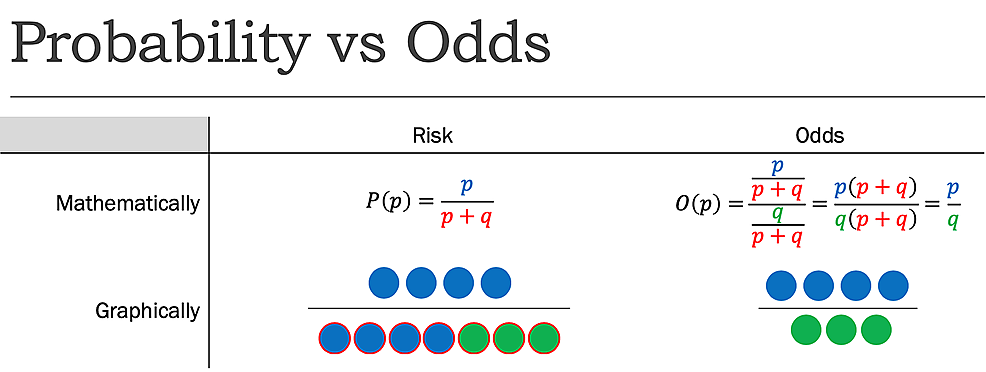## 5.3 Probabilities

A logistic regression model allows us to establish a relationship between a binary outcome variable and a group of predictor variables. It models the logit transformed probability as a linear relationship with the predictor variables. More formally, let $$Y$$ be the binary outcome variable indicating failure/success with $$\{0,1\}$$ and $$p$$ be the probability of $$y$$ to be $$1, p=P(Y=1)$$. Let $$x_{1}, \cdots, x_{k}$$ be a set of predictor variables. Then the logistic regression of $$Y$$ on $$x_{1}, \cdots, x_{k}$$ estimates parameter values for $$\beta_{0}, \beta_{1}, \cdots, \beta_{k}$$ via maximum likelihood method of the following equation $\operatorname{logit}(\pi)=\log \left(\frac{\pi}{1-\pi}\right)=\beta_{0}+\beta_{1} x_{1}+\cdots+\beta_{k} x_{k} .$ Exponentiate and take the multiplicative inverse of both sides, $\frac{1-\pi}{\pi}=\frac{1}{\exp \left(\beta_{0}+\beta_{1} x_{1}+\cdots+\beta_{k} x_{k}\right)} .$ Partial out the fraction on the left-hand side of the equation and add one to both sides, $\frac{1}{\pi}=1+\frac{1}{\exp \left(\beta_{0}+\beta_{1} x_{1}+\cdots+\beta_{k} x_{k}\right)} .$ Change 1 to a common denominator, $\frac{1}{\pi}=\frac{\exp \left(\beta_{0}+\beta_{1} x_{1}+\cdots+\beta_{k} x_{k}\right)+1}{\exp \left(\beta_{0}+\beta_{1} x_{1}+\cdots+\beta_{k} x_{k}\right)}$ Finally, take the multiplicative inverse again to obtain the formula for the probability $$P(Y=1)$$, $\pi=\frac{\exp \left(\beta_{0}+\beta_{1} x_{1}+\cdots+\beta_{k} x_{k}\right)}{1+\exp \left(\beta_{0}+\beta_{1} x_{1}+\cdots+\beta_{k} x_{k}\right)} .$

• Probability: if the probability of success is $$\pi=0.8$$, the probability of failure is $$1-\pi=0.2$$

• Odds: It is the ratio between the probability of success and the probability of failure. $$\operatorname{odds}= \dfrac{\pi}{1-\pi}=\dfrac{0.8}{0.2}=4.$$ That is, the odds of success are 4 to 1.

• Odds Ratio: It is the ratio between Odds. $$\operatorname{OR}= \dfrac{\operatorname{odds}_1}{\operatorname{odds}_2}=\dfrac{\frac{\pi_1}{1-\pi_1}}{\frac{\pi_2}{1-\pi_2}}$$